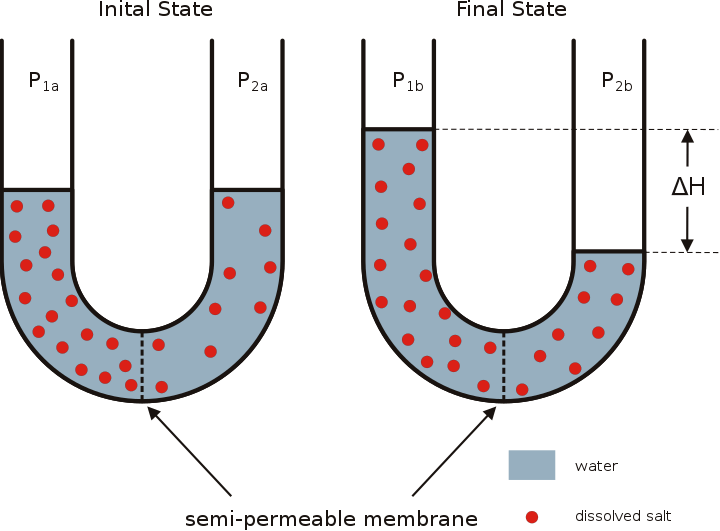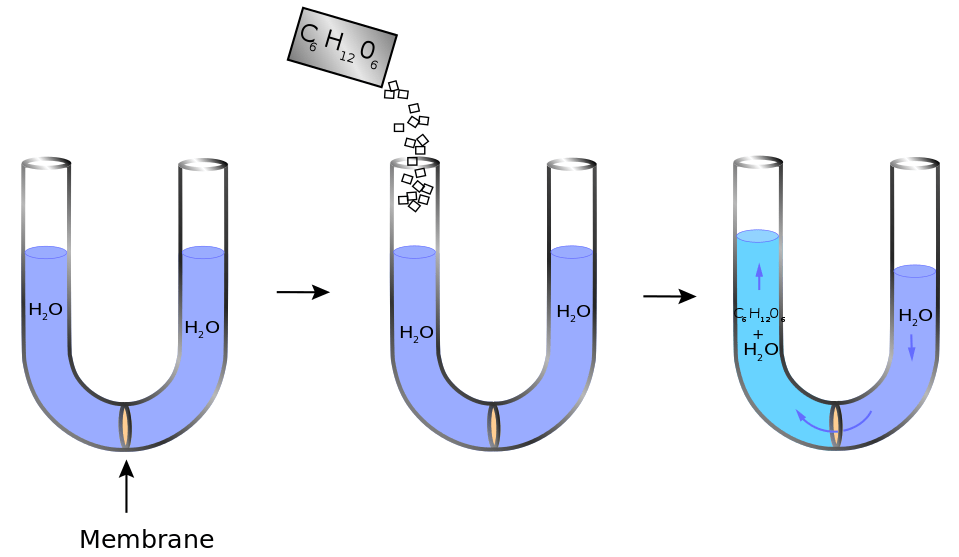# Difference Between Osmolarity and Osmolality

## Main Difference – Osmolarity vs Osmolality

Osmotic pressure is a term that concerns fluids. It is the pressure that is needed to avoid osmosis. In other words, osmotic pressure is the pressure that has to be applied in order to avoid a pure solution entering a solution having dissolved solutes through osmosis. Osmolarity and osmolality are connected to osmotic pressure. They are used to measure the osmotic pressure in terms of osmoles. An osmole is the number of moles in a compound that contributes to the osmotic pressure. The main difference between osmolarity and osmolality is that osmolarity is a measure considering the volume of a solution whereas osmolality is measured considering the mass of a solution.

### Key Areas Covered

1. What is Osmolarity
– Definition, Explanation with Examples
2. What is Osmolality
– Definition, Explanation with Examples
3. What is the Relationship Between Osmolarity and Osmolality
– Explanation of Equation
4. What is the Difference Between Osmolarity and Osmolality
– Comparison of Key Differences

Key Terms: Osmolality, Osmolarity, Osmoles, Osmotic Pressure## What is Osmolarity

Osmolarity is the number of osmoles per liter of a solution that is being considered. In other words, it is a term used to express the concentration of a solution that shows osmotic activity. This means Osmolarity is the concentration of an osmotic solution. This is also called osmotic concentration.Figure 1: Osmosis

Unlike molarity, osmolarity measures the number of particles per liter. For example, if we consider a NaCl solution, the osmolarity is given as twice as the molarity of that solution. This is because the molarity is the number of Na or Cl moles per liter whereas osmolarity is (Na+Cl) moles per liter since all the particles are considered.

If we consider a solution of an ionic compound, all the ions are considered as particles rather than particular ions. A particle can be either an ion or a molecule. But if we consider a solution of a compound having a molecular structure, then the compound will dissolve forming molecules. Then each molecule is considered as a particle.

For example,

• If 0.08 moles of glucose is dissolved in 1 liter of water;

Molarity of glucose solution =   0.08 mol/L

Osmolarity of glucose solution =   0.08 osmol/L

• If 0.08 moles of NaCl is dissolved in 1 liter of water;

Molarity of NaCl solution =   0.08 mol/L

Osmolarity of NaCl solution =   0.16 osmol/L

Osmolarity does not depend on the type of compound that is dissolved in the solution. It just measures the amount of particles present there. But it is affected by the changes in the solvent (water). That is because if the volume of solution is increased or decreased, the volume considered for the calculation of osmolarity is changed accordingly. Moreover, osmolarity also depends on the temperature and pressure since the volume and solubility of compounds can be changed by changing those parameters.

## What is Osmolality

Osmolality is a term used to describe the osmotic pressure of a solution regarding the solution mass. It can be defined as the number of osmoles per one kilogram of the solution. The osmoles are the number of moles of particles present in that solution. The particles can be either ions or molecules.

Since the mass is independent of pressure and temperature, these two parameters cannot affect the osmolality of a solution. But a change in water content can highly affect osmolality since it changes the mass of the solution. This characteristic is advantageous in the determination of osmolality accurately at any temperature and pressure condition.

Osmolality can easily be determined by freezing point depression method. It is based on the indirect proportionality of solutes and the freezing point of the solution (the more solute in a solution, the lower will be its freezing point)Figure 2: The osmotic pressure arises when a compound is dissolved in water.

The unit for osmolality is osmol/kg. Osmolality gives the concentration of a solute in a solution in terms of the mass of the solution.

## Relationship Between Osmolarity and Osmolality

• For very dilute solutions, osmolarity and osmolality are numerically the same.
• The difference between osmolality and osmolarity is called osmolar gap.

## Difference Between Osmolarity and Osmolality

### Definition

Osmolarity: Osmolarity is the concentration of a solution expressed as osmoles of solute particles per liter of solution.

Osmolality: Osmolality is the concentration of a solution expressed as the total number of solute particles per kilogram.

### Calculation

Osmolarity: Osmolarity is calculated considering the volume of solution.

Osmolality: Osmolality is calculated considering the mass of solution

### Units

Osmolarity: Units for osmolarity is mol/L.

Osmolality: Units for osmolality is osmol/L.

### Temperature and Pressure

Osmolarity: Osmolarity depends on the temperature and pressure.

Osmolality: Osmolality does not depend on the temperature and pressure.

### Conclusion

Both osmolarity and osmolality are used to determine the osmotic pressure of a system. They describe the concentration of a solution in terms of osmoles. However, they have some differences as discussed above in this article. The main difference between osmolarity and osmolality is that osmolarity is measured considering the volume of a solution whereas osmolality is measured considering the mass of a solution.

##### References:

1. Canon, Martin. “Osmoles, , Osmolality and osmotic pressure: Clarifying the puzzle of solution concentration.” PubMed (2008): 92-99. Researchgate.net. Researchgate.net, June 2008. Web. Available here. 11 Aug. 2017.
2. “Osmotic concentration.” Wikipedia. Wikimedia Foundation, 13 July 2017. Web. Available here. 11 Aug. 2017.

##### Image Courtesy:

1. “Osmose en” By © Hans Hillewaert (CC BY-SA 3.0) via Commons Wikimedia
2. “Osmosis diagram” By KDS4444 – Own work (CC0) via Commons Wikimedia